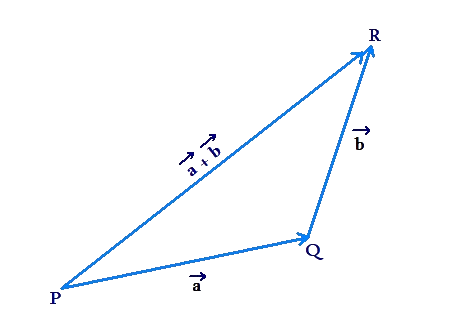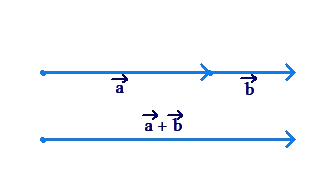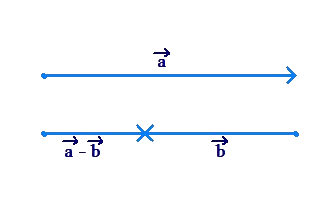# Triangle Inequality in Vectors

Triangle Inequality in Vectors

The following figure shows a triangle which is formed by the vectors $$\vec a$$, $$\vec b$$, and $$\vec a\, + \vec b$$:From plane geometry, we know that in any triangle, the sum of two sides is greater than the third side. In the figure above, PQ = $$\left| {\vec a} \right|$$, QR = $$\left| {\vec b} \right|$$ and PR = $$\left| {\vec a\, + \vec b} \right|$$. Thus,

$\left| {\vec a\, + \vec b} \right| < \left| {\vec a} \right| + \left| {\vec b} \right| ...\left( i \right)$

Also, we know that the difference of two sides in a triangle is less than the third side. The difference of two quantities x and y can be written as $$\left| {x - y} \right|$$. We take the modulus sign because we don’t know which quantity is larger: x or y; and when we say difference, we mean the positive difference. For example, the difference of 3 and 5 is $$\left| {3 - 5} \right| = 2$$. Coming back to our triangle, the difference of PQ and QR can be written as |PQ $$-$$ QR|. Thus,

$\begin{array}{l}\left| {{\rm{PQ}} - {\rm{QR}}} \right| < \left| {{\rm{PR}}} \right|\\ \Rightarrow \,\,\,\left| {\left| {\vec a} \right| - \left| {\vec b} \right|} \right| < \left| {\vec a\, + \vec b} \right| & ...\left( {ii} \right)\end{array}$

Combining (i) and (ii), we have the triangle inequality for vectors:

$\underbrace {\left| {\left| {\vec a} \right| - \left| {\vec b} \right|} \right|}_{\scriptstyle{\rm{Difference\;of }}\atop\scriptstyle{\rm{two\;sides}}} < \underbrace {\left| {\vec a\, + \vec b} \right|}_{{\rm{Third\;side}}} < \underbrace {\left| {\vec a} \right| + \left| {\vec b} \right|}_{\scriptstyle{\rm{Sum\;of\; }}\atop\scriptstyle{\rm{two \;sides}}}$

Don’t get intimidated by the seemingly complicated look of this inequality. It is simply an expression of the fact that any side in a triangle is less than the sum of the other two sides, and greater than their difference. In this case, the side $$\left| {\vec a\, + \vec b} \right|$$ is less than the sum of the other two sides $$\left| {\vec a} \right| + \left| {\vec b} \right|$$, and greater than their difference $$\left| {\left| {\vec a} \right| - \left| {\vec b} \right|} \right|$$.

What if the vectors $$\vec a$$ and $$\vec b$$ are parallel (in the same direction), as shown in the figure below?In this case, $$\left| {\vec a\, + \vec b} \right|$$ will be equal to $$\left| {\vec a} \right| + \left| {\vec b} \right|$$. Make sure you understand this.

The other special case is when vectors $$\vec a$$ and $$\vec b$$ are anti-parallel (in the opposite directions), as shown below:In this case, $$\left| {\vec a\, + \vec b} \right|$$ is equal to the difference in the magnitudes of the two vectors, or $$\left| {\left| {\vec a} \right| - \left| {\vec b} \right|} \right|$$.

If we incorporate these two special cases, we have the complete version of the triangle inequality:

$\left| {\left| {\vec a} \right| - \left| {\vec b} \right|} \right| \le \left| {\vec a\, + \vec b} \right| \le \left| {\vec a} \right| + \left| {\vec b} \right|$

grade 9 | Questions Set 1